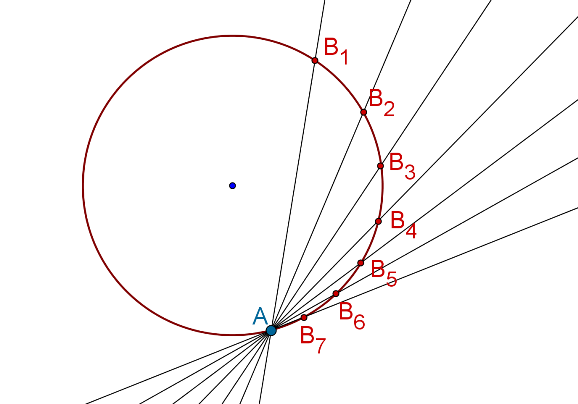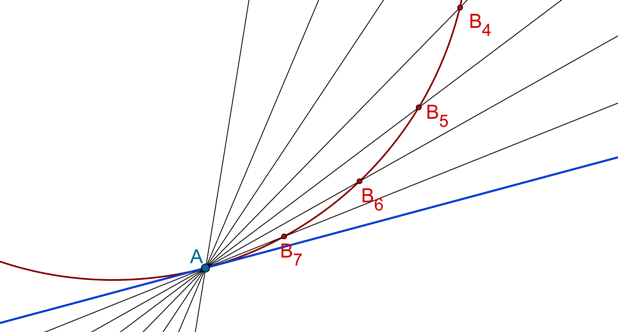# Tangent as a Special Case of Secant

Tangent as a Special Case of Secant

We have seen that a secant for a circle intersects the circle in two points. Let L be a secant to a circle S which intersects S at A and B. What would happened if A and B were really close to each other? In the following figure, the position of A is fixed, but B is moved and brought closer and closer to A. What happens to the secant L?Different positions of the moving point B have been marked with different subscripts: B1, B2, and so on. As this point comes closer to A, note how the secant comes closer and closer to the tangent at A. Let us zoom in on the region around A. We have highlighted the tangent at A. Note how the secant approaches the tangent as B approaches A:Thus (and this is really important): we can think of a tangent to a circle as a special case of its secant, where the two points of intersection of the secant and the circle coincide. This insight will prove to be really useful when you study the subject of Calculus in higher classes.

Circles and Tangents
grade 10 | Questions Set 1
Circles and Tangents